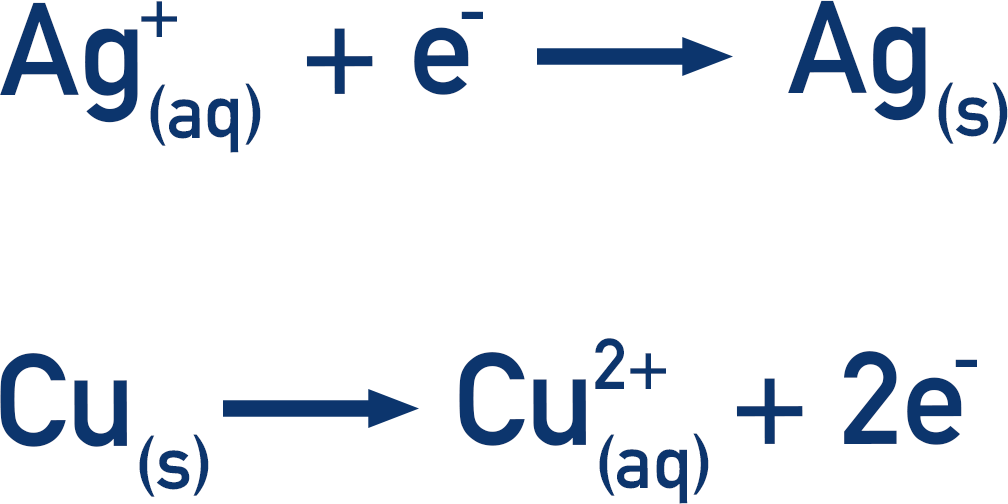Quick Notes Electrode Potentials

• The relative potentials of half-cells are found by comparing them all to the same type of half-cell. The half-cell used is called the standard hydrogen electrode.
• If two hydrogen half-cells (standard hydrogen electrodes) are connected, the potential difference of the electrochemical cell = 0V.
• If one of the half-cells is changed to a different type of half-cell, there will be a difference in potential between the two – enabling a comparison to be made.
• All potential differences measured are referred to as standard electrode potentials.
• For an electrode potential to be considered standard, all ions in solution for the half-cell being used must be 1 mol dm-3, temperature must be 298K and, for gaseous half-cells, the pressure must be 101kPa (standard conditions).
• The potential difference for a cell (Ecell) made of two different half-cells can be calculated by finding the difference between their standard electrode potentials.• In an electrochemical cell the cathode is the electrode where reduction takes place, the anode is the electrode where oxidation takes place. So, finding the Ecell potential difference can also be written as:Full Notes Electrode Potentials

It’s the relative difference in electrode potentials of half-cells that’s important in electrochemistry – how they compare to one another.

If an electrochemical cell is made using two half-cells that are the same, the difference in potential between them will obviously be zero (0V).

If one of those half-cells is changed, there will be a difference in potential, enabling a relative comparison of electrode potentials to be made.

By taking a standard hydrogen electrode and connecting it to different half-cells (under standard conditions), a range of potential differences can be measured. The potential difference measured is called a standard electrode potential.

Note – For an electrode potential to be considered standard, all ions in solution for the half-cell being used must be 1 mol dm-3, temperature must be 298K and, for gaseous half-cells, the pressure must be 101kPa (standard conditions).

The electrochemical series is a range of standard electrode potentials for different half-cells arranged in order. These values given are also sometimes referred to as ‘redox potentials’ (see below!).

Half Reaction (from a half-cell) Standard Potential
Li+(aq)+ e- ⇌ Li(s) -3.05V
Mg2+(aq)+ 2e- ⇌ Mg(s) -2.37V
Al3+(aq)+ 3e- ⇌ Al(s) -1.66V
Zn2+(aq)+ 2e- ⇌ Zn(s) -0.76V
Pb2+(aq)+ 2e- ⇌ Pb(s) -0.13V
2H+(aq)+ 2e- ⇌ H2(g) 0.00V
Cu2+(aq)+2e- ⇌ Cu(s) +0.34V
Fe2+(aq)+ 2e- ⇌ Fe(s) +0.44V
Fe3+(aq)+e- ⇌ Fe2+(aq) +0.77V
Ag+(aq)+ e- ⇌ Ag(s) +0.80V
O2(g)+ 4H+(aq)+ 4e- ⇌ 2H2O(l) +1.23V
Cl(g)+ 2e- ⇌ 2Cl-(aq) +1.36V
Au+(aq)+ e- ⇌ Au(s) +1.69V

This table can look very confusing at first. The standard potentials shown are the reading on a voltmeter, when each half-cell is connected to the standard hydrogen electrode.

We now have an easy way to compare the half reactions occurring. Remember, the more negative the value for a standard potential, the more likely the reaction is to happen in the reverse direction (oxidation). The more positive the value, the more likely the reaction is to happen in the forwards direction (reduction).

This means we can predict what will happen when two half-cells are connected. The reaction in the half-cell with the more positive standard potential will happen in the forward direction (reduction); the reaction in the half-cell with the more negative standard potential will happen in the reverse direction (oxidation).

For example, if we connected the following two half-cells:

Ag+(aq)+ e-→Ag(s) +0.80V

Cu2+(aq)+2e-→Cu(s) +0.34V

The Ag/Ag+ half-cell is more positive than the Cu/Cu2+ half-cell. This means the reaction in the Ag/Ag+ half-cell will go forwards (reduction). So, the reaction in the Cu/Cu2+ half-cell must go in the reverse direction (oxidation).

This now means the following reactions occur:The Cu(s) is oxidised, so the released electrons go to the other half-cell and reduce the Ag+(aq)ions. This movement of electrons means there is a flow of electricity. Note, ions also move through the salt bridge to complete the circuit, but no further knowledge of this is required for A-level Chemistry.

To calculate the potential difference of the cell (Ecell), we simply need to find the difference between the standard electrode potentials for each half-cell.

This gives us the equation:Using the values in the table above:

Ecell = (+0.80) – (+0.34) = 0.46V

You may see this as:The most positive half-cell undergoes reduction and the most negative half-cell undergoes oxidation, hence the above way of writing the equation.

There are different ways of writing these equations. It is rare at A-level, but sometimes you may see it written as the following.This is because the anode is the electrode where oxidation takes place, and the cathode is the electrode is where reduction takes place.